# Non Verbal Reasoning - Water Images

Exercise : Water Images - Section 2
Directions to Solve

In each of the following questions, choose the water image of the Fig.(X) from amongst the four alternatives (1), (2), (3) and (4) given along with it.

1.

Choose the correct water image of the given figure (X) from amongst the four alternatives.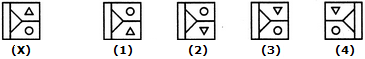1
2
3
4
Explanation:
No answer description is available. Let's discuss.

2.

Choose the correct water image of the given figure (X) from amongst the four alternatives.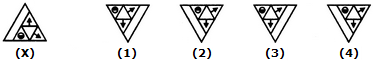1
2
3
4
Explanation:
No answer description is available. Let's discuss.

3.

Choose the correct water image of the given figure (X) from amongst the four alternatives.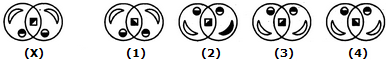1
2
3
4
Explanation:
No answer description is available. Let's discuss.

4.

Choose the correct water image of the given figure (X) from amongst the four alternatives.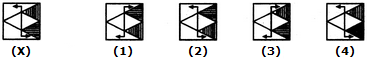1
2
3
4
Explanation:
No answer description is available. Let's discuss.

5.

Choose the correct water image of the given figure (X) from amongst the four alternatives.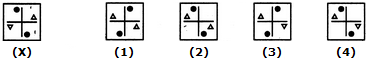1
2
3
4